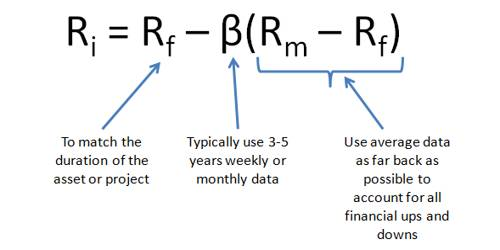# Capital Asset Pricing Model (CAPM)

The capital asset pricing model (CAPM) provides a formula that calculates the expected return on a security based on its level of risk. The formula for the capital asset pricing model is the risk-free rate plus beta times the difference of the return on the market and the risk-free rate.

CAPM Model

This model presents a very simple theory that delivers a simple result. The theory says that the only reason an investor should earn more, on average, by investing in one stock rather than another is that one stock is riskier. It is widely used throughout finance for pricing risky securities and generating expected returns for assets given the risk of those assets and cost of capital. Not surprisingly, the model has come to dominate modern financial theory. But does it really work?

It’s not entirely clear. The big sticking point is beta. When Professors Eugene Fama and Kenneth French looked at share returns on the New York Stock Exchange, the American Stock Exchange and Nasdaq between 1963 and 1990, they found that differences in betas over that lengthy period did not explain the performance of different stocks. The linear relationship between beta and individual stock returns also breaks down over shorter periods of time.

CAPM is calculated according to the following formula:

Ra = Rrf + [Ba x (Rm – Rrf)]

Where:

• Ra = Expected return on a security
• Rrf = Risk-free rate
• Ba = Beta of the security
• Rm = Expected return of the market

Note: “Risk Premium” = (Rm – Rrf)

The CAPM formula is used to calculate the expected return on investable asset. The formula for the capital asset pricing model is the risk-free rate plus beta times the difference of the return on the market and the risk-free rate.